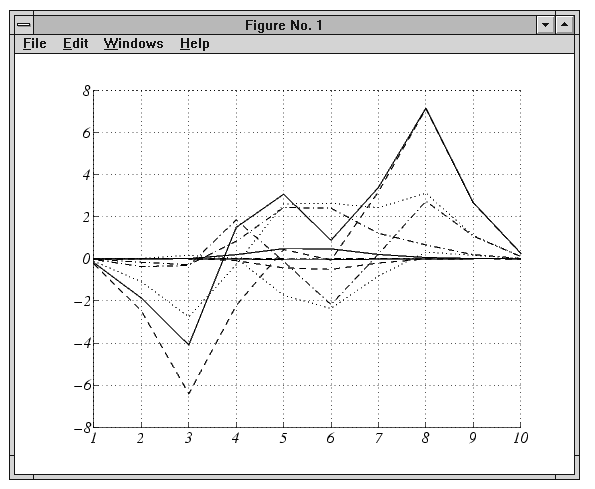GraphicsExample -- Using newplot

To illustrate the use of `newplot`, this example creates a function that is similar to the built-in `plot` function, except it automatically cycles through different line styles instead of using different colors for multiline plots.

• ```function my_plot(x,y)
cax = newplot; % ```newplot returns handle of current axes
```LSO = ['`-` ';'`--`';': ';'`-`.'];
set(cax,'FontName','Times','FontAngle','italic')
set(get(cax,'Parent'),'MenuBar','none') %
line_handles = line(x,y,'Color','b');
style = 1;
for i = 1:length(line_handles)
if style > length(LSO), style = 1;end
set(line_handles(i),'LineStyle',LSO(style,:))
style = style + 1;
end
grid on
```

The function `my_plot` uses the high-level `line` function syntax to plot the data. This provides the same flexibility in input argument dimension that the built-in `plot` function supports. The `line` function does not check the value of the figure or axes `NextPlot` property. However, because `my_plot` calls `newplot`, it behaves the same way the high-level `plot` function does -- with default values in place, `my_plot` clears and resets the axes each time you call it.

`my_plot` uses the handle returned by `newplot` to access the target figure and axes. This example sets axes font properties and disables the figure's menu bar. Note how the figure handle is obtained via the axes `Parent` property.

This picture shows typical output for the `my_plot` function.

• ```my_plot(1:10,peaks(10))```

Basic Plotting M-File Structure

This example illustrates the basic structure of graphics M-files:

• Call `newplot` early to conform to the `NextPlot` properties and to obtain the handle of the target axes.
• Reference the axes handle returned by `newplot` to set any axes properties or to obtain the figure's handle.
• Call object creation functions to draw graphics objects with the desired characteristics.

The MATLAB default settings for the `NextPlot` properties facilitate writing M-files that adhere to the standard behavior: reuse the figure window, but clear and reset the axes with each new graph. Other values for these properties allow you to implement different behaviors.

Replacing Only the Child Objects -- replacechildren

The `replacechildren` value for `NextPlot` causes `newplot` to remove child objects from the figure or axes, but does not reset any property values (except the list of handles contained in the `Children` property).

This can be useful after setting properties you want to use for subsequent graphs without having to reset properties. For example, if you type on the command line

• ```set(gca,'ColorOrder',[0 0 1],'LineStyleOrder','`-`|`--`|:|`-`.',...
'NextPlot','replacechildren')
plot(x,y)
```

`plot` produces the same output as the M-file `my_plot` in the previous section, but only within the current axes. Calling `plot` still erases the existing graph (i.e., deletes the axes children), but it does not reset axes properties. The values specified for the `ColorOrder` and `LineStyleOrder` properties remain in effect.Targeting Graphics Output with newplot Testing for Hold State© 1994-2005 The MathWorks, Inc.